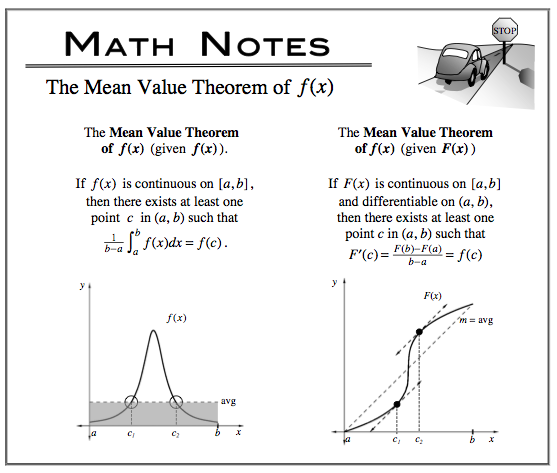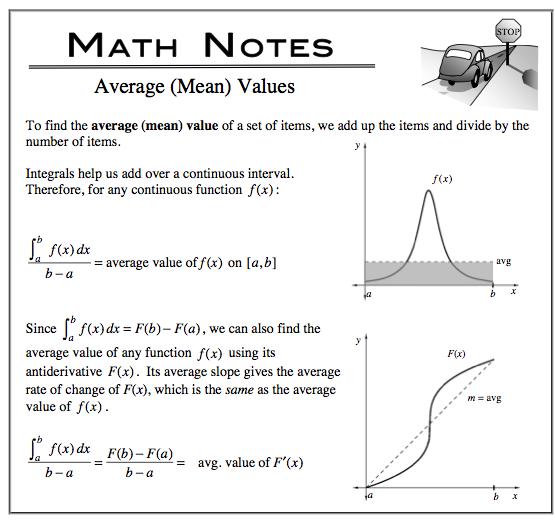### Home > CALC > Chapter 7 > Lesson 7.1.4 > Problem7-45

7-45.
1. Kimberly and Varag are in a bicycle race. Homework Help ✎

1. If Kimberly's velocity in miles per hour during the race is v(t) = 2.3t sin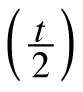, find the bicyclist's average velocity during 0 ≤ t ≤ 6. Describe your method.

2. If Varag's distance from the starting line during the race is s(t) = 20t + 2 sin(t), find his average velocity during 0 ≤ t ≤ 6. Describe your method.

3. When is each bicyclist traveling at his/her average velocity?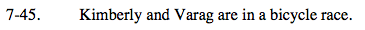For parts (a) and (b), each is a different application of Mean Value. Pay attention to the given information and read carefully.
See Math Notes below.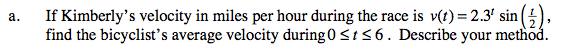Average Velocity = 16.102 mph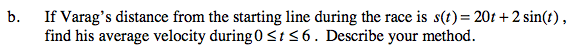Average Velocity = 19.907 mph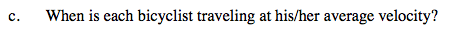This is an application of Mean Value Theorem, which states that a function (such as a velocity function) must equal its average value at least once on a closed interval (that is, if the function is continuous and differentiable).
See Math Notes below.